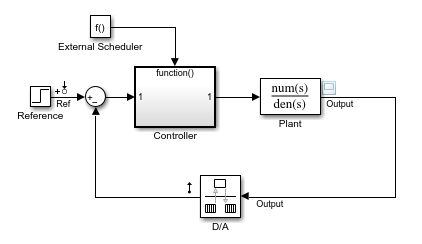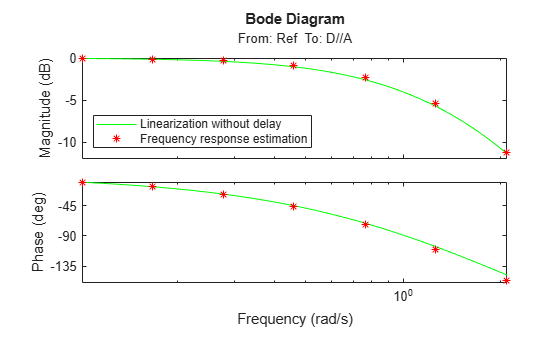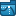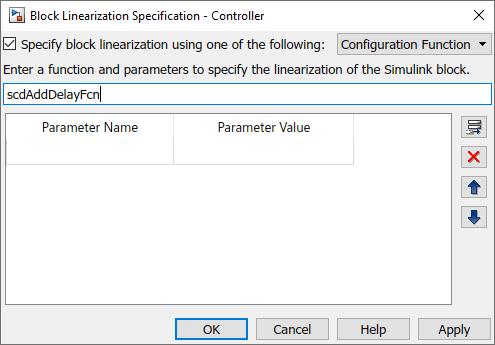# Augment Block Linearization

This example shows how to augment the linearization of a block with additional time delay dynamics using a block linearization specification function.

Open the Simulink model.

```mdl = 'scdFcnCall'; open_system(mdl)```This model includes a continuous-time plant and a discrete-time controller. The D/A block discretizes the plant output with a sample time of 0.1 s. The External Scheduler block triggers the controller to execute with the same period. However, the trigger has an offset of 0.05 s relative to the discretized plant output. For that reason, the controller does not process a change in the reference signal until 0.05 s after the change occurs. This offset introduces a time delay of 0.05 s into the model.

Linearize the closed-loop model at the model operating point without specifying a linearization for the Controller block.

```io = getlinio(mdl); sys_nd = linearize(mdl,io);```

Check the linearization result by frequency response estimation.

```input = frest.Sinestream(sys_nd); sysest = frestimate(mdl,io,input); bode(sys_nd,'g',sysest,'r*',{input.Frequency(1),input.Frequency(end)}) legend('Linearization without delay',... 'Frequency response estimation','Location','southwest')```The exact linearization does not account for the time delay introduced by the controller execution offset. There is a discrepancy in the results between the linearized model and the estimated model, especially at higher frequencies.

Create a function to specify the linearization of the Controller block that includes the time delay. For this example use the linearization specified in `scdAddDelayFcn.m`.

```function sys = scdAddDelayFcn(BlockData) sys = BlockData.BlockLinearization*thiran(0.05,0.1); end ```

The input to the function, `BlockData`, is a structure that the software creates automatically each time it linearizes the block. When you specify a block linearization configuration function, the software automatically passes `BlockData` to the function. The field `BlockLinearization` contains the current linearization of the block.

This configuration function approximates the time delay as a Thiran filter. The filter indicates a discrete-time approximation of the fractional time delay of 0.5 sampling periods. (The 0.05 s delay has a sample time of 0.1 s).

Specify `scdAddDelayFcn` as the linearization for the Controller block.

1. Right-click the Controller block, and select Linear Analysis > Specify Selected Block Linearization.

2. In the Block Linearization Specification dialog box, select Specify block linearization using one of the following.

3. In the drop-down list, select `Configuration Function`.

4. In the text box, enter the function name `scdAddDelayFcn`. This function has no additional parameters, so leave the parameter table blank.

5. Click OK.Alternatively, you can specify the configuration function programmatically using the following code.

```block = 'scdFcnCall/Controller'; set_param(block,'SCDEnableBlockLinearizationSpecification','on') rep = struct('Specification','scdAddDelayFcn',... 'Type','Function',... 'ParameterNames','',... 'ParameterValues',''); set_param(block,'SCDBlockLinearizationSpecification',rep)```

Linearize the model using the augmented block linearization.

`sys_d = linearize(mdl,io);`

Compare the linearization that includes the delay with the estimated frequency response.

```bode(sys_d,'b',sys_nd,'g',sysest,'r*',... {input.Frequency(1),input.Frequency(end)}) legend('Linearization with delay','Linearization without delay',... 'Frequency response estimation','Location','southwest')```The linear model obtained using the augmented block linearization now accounts for the time delay. This linear model more closely matches the real frequency response of the model.